Five tractors

Five tractors plow field in 4.8 hours. How long plow this field if after 1.5 hours of work two tractors went to a different field?

Result

x =  7 h

Solution:1.5*5/(4.8*5) + (x-1.5)*3/(4.8*5)=1

0.125x = 0.875

x = 7

Calculated by our simple equation calculator.

Leave us a comment of this math problem and its solution (i.e. if it is still somewhat unclear...):Be the first to comment!To solve this verbal math problem are needed these knowledge from mathematics:

Do you have a linear equation or system of equations and looking for its solution? Or do you have quadratic equation?

Next similar math problems:

1. Welders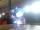Welder do the job for six hours, the second one for 8 hours. How long do the job third welder if all together can do job in 2 hours?
2. Workers 2Worker dug the trench for 7 hours, another worker for 10 hours. They recruited to work even one worker more. Digging should be done for 2 hours. For how long would dig third worker alone?
3. Two tributaries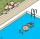Two tributaries of the pool fill it in 10 hours. One of the tributaries would fill 15 hours. How long would fill the first tributary?
4. Painters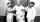One painter could paint a school for 7 days. The second one for 6 days. It was necessary to paint the school in two days. Next painter was invited and all worked together. How long would this painter painted the school itself?
5. Hectoliters of waterThe pool has a total of 126 hectoliters of water. The first pump draws 2.1 liters of water per second. A second pump pumps 3.5 liters of water per second. How long will it take both pumps to drain four-fifths of the water at the same time?
6. Simply equationSolve this equation for x: ?
7. Painting school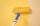Redecoration school had scheduled for four crew to 10 days. After two days a single worker ill. For how many days the school was painted when remain workers working with the same tempo?
8. 12 Moons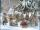Good Marry came to ask twelve moons for help with collecting strawberries. All twelve moons 1200 strawberries picked in 20 minutes. But lazy months July and August cease tear after 5 minutes. How many minutes in total will take collect strawberries?
9. Liquid soap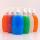Liquid soap lasted two weeks to one member of the family. How many days will last, if it use: a) two members b) 4 members c) seven members?
10. Collection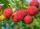John has collected half a bucket of raspberries in 45 minutes, Eva collects the whole bucket in an hour. How many minutes it would take to fill a bucket if the two children work together?
11. BicycleThe bicycle pedal gear has 36 teeth, the rear gear wheel has 10 teeth. How many times turns rear wheel, when pedal wheel turns 120x?
12. Simple equationSolve for x: 3(x + 2) = x - 18
13. CandyPeter had a sachet of candy. He wanted to share with his friends. If he gave them 30 candies, he would have 62 candies. If he gave them 40 candies, he would miss 8 candies. How many friends did Peter have?
14. Fifth of the numberThe fifth of the number is by 24 less than that number. What is the number?
15. EquationSolve the equation: 1/2-2/8 = 1/10; Write the result as a decimal number.
16. Reciprocal equationDetermine the root of the equation: 9/x-7/x=1
17. Unknown numberIdentify unknown number which 1/5 is 40 greater than one tenth of that number.